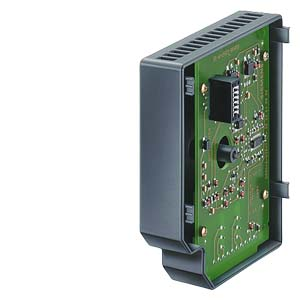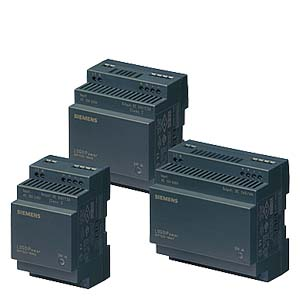• 资质认证 实名商家

##关于我们
• 企业文化 组织结构 分支公司 售后服务 技术支持
•••• 产品分类

## V90•••4. 采用高级语言编写部分重要的工艺程序

 在项目具体实施的过程中，我们应该从软件开发技巧的度来考虑PLC控制程序的保护：

1. 编程方式的采用
a) 采用模块化的程序结构，采用符号名，参数化来编写子程序块
b) S7-300/400尽量采用背景数据块和多重背景的数据传递方式
c) 多采用间接寻址的编程方式
d) 复杂系统的控制程序尤其是一些带有顺序控制或配方控制的程序，可以考虑采用数据编程的方式，即通过数据的变化来改变系统的控制逻辑或控制顺序。

2. 主动保护方法
a) 利用系统的时钟
b) 利用程序卡或者CPU的ID号和序列号
c) 利用EEPROM的反写入功能，及一些需要设置的内存保持功能
d) 利用系统提供的累时器功能
e) 在用户程序的数据块中设置密码
f) 软件上设置逻辑陷阱
g) 可以反向利用自己在编程时犯的错误

3. 被动保护方法
a) 在内存容量利用许可的条件下，不要删除被认为是无用的程序
b) 在数据块里留下开发者的标识，以便于将来遭到侵权时可以取证

4. 应用反技术的注意事项
a) 在用户程序中嵌入保护程序要显得自然一些，不能很突兀的加出一段程序来，代码要尽量精简，变量符号名应与被嵌入程序段的变量保持一致
b) 往往一种保护加密手段是不够的，应该多种方法并用，并且这些保护程序一旦后对系统造成的后果也应该尽量不同，造成所谓的“效应”，从而增加程序被的难度，时间与成本，短时间内让者束手无策，
c) 保护好程序的原代码，如果需要交付程序的，在不影响用户对设备维护的前提下，应对交付的程序做适当的技术处理，如删除部分符号名，采用上载的程序或数据块
d) 做好严格的测试，以避免保护程序的不完善引起的误动作而带来的不必要的麻烦，同时也能降低售后服务的的费用

 运用保护手段的原则

*后，这仅仅只是一个测试程序，如果各位想用到商业方面，还需要各位自己去完善。

///////////////////////////////////////

TITLE=程序注释

Network 1 // 网络标题

// 在第一个循环周期内初始化Modbus从站协议

LD SM0.1

CALL SBR3, 1, 12, 9600, 0, 0, 128, 32, 1000, &VB0, M10.1, MB11

Network 2

// 在每个循环周期内执行Modbus 从站协议

LD SM0.0

CALL SBR1, M10.2, MB12

Network 3

LD SM0.0

MOVW AIW0, VW100

/I +54, VW100

MOVW AIW2, VW102

/I +54, VW102

MOVW AIW4, VW104

/I +54, VW104

MOVW AIW6, VW106

/I +54, VW106

Network 4

LD SM0.0

= Q0.0

Network 5

LD I0.0

= Q0.1

Network 6

LD I0.1

= Q0.2

///////////////////////////////////

Option Explicit

Private Declare Function GetTickCount Lib "kernel32" () As Long

Dim x1

Dim p11, p12, p13, p14, p15, p16, p17, p18

Function CRC16(data() As Byte) As bbbbbb

Dim CRC16Lo As Byte, CRC16Hi As Byte 'CRC寄存器

Dim CL As Byte, CH As Byte '多项式码&HA001

Dim SaveHi As Byte, SaveLo As Byte

Dim i As Integer

Dim Flag As Integer

CRC16Lo = &HFF

CRC16Hi = &HFF

CL = &H1

CH = &HA0

For i = 0 To UBound(data)

CRC16Lo = CRC16Lo Xor data(i) '每一个数据与CRC寄存器进行异或

For Flag = 0 To 7

SaveHi = CRC16Hi

SaveLo = CRC16Lo

CRC16Hi = CRC16Hi \ 2 '高位右移一位

CRC16Lo = CRC16Lo \ 2 '低位右移一位

If ((SaveHi And &H1) = &H1) Then '如果高位字节*后一位为1

CRC16Lo = CRC16Lo Or &H80 '则低位字节右移后补1

End If '否则自动补0

If ((SaveLo And &H1) = &H1) Then '如果LSB为1，则与多项式码进行异或

CRC16Hi = CRC16Hi Xor CH

CRC16Lo = CRC16Lo Xor CL

End If

Next Flag

Next i

Dim ReturnData(1) As Byte

ReturnData(0) = CRC16Hi 'CRC高位

ReturnData(1) = CRC16Lo 'CRC低位

CRC16 = ReturnData

End Function

End Sub

Timer1.Enabled = False '定时器1无效

Timer1.Interval = 1000 '定时器1时间为1S

Timer2.Enabled = True '定时器2有效

Timer2.Interval = 1000 '定时器2时间为1S

Text1.Text = ""

Text2.Text = ""

Text3.Text = ""

Text4.Text = ""

Text5.Text = ""

Text6.Text = ""

Text8.Text = ""

Text10.Text = ""

mPort = 1 '设定端口号

MSComm1.Settings = "9600,n,8,1" '设定通讯波特率

MSComm1.InBufferSize = 1024 '接收缓冲器大小

MSComm1.OutBufferSize = 1024 '输出缓冲器大小

MSComm1.bbbbbMode = combbbbbModeBinary '以二进制传输

MSComm1.RThreshold = 1 '

MSComm1.SThreshold = 0

MSComm1.bbbbbLen = 0 '读取接收缓冲器所有字符

MSComm1.OutBufferCount = 0 '清空发送缓冲区

MSComm1.InBufferCount = 0 '清空接收缓冲区

If MSComm1.PortOpen = False Then

Command3.Caption = "打开串口"

Else

Command3.Caption = "关闭串口"

End If

End Sub

Private Sub Command1_Click()

Timer1.Enabled = True

End Sub

Private Sub Command2_Click() '退出程序，定时器1无效

Timer1.Enabled = False

Cls

End Sub

Private Sub Command3_Click()

On Error Resume Next

If MSComm1.PortOpen = False Then

MSComm1.PortOpen = True

Else

MSComm1.PortOpen = False

End If

If MSComm1.PortOpen Then '打开关闭按钮显示文字

Command3.Caption = "关闭串口"

Else

Command3.Caption = "打开串口"

End If

If Err Then '打开串口失败，则显示出错信息

MsgBox Error\$, 48, "错误信息"

Exit Sub

End If

End Sub

Private Sub Timer1_Timer() '读V存储区数据

Dim CRC() As Byte

Dim FGetData As bbbbbb

Dim aa() As Byte

Dim s As bbbbbb

Dim str As bbbbbb

Dim i As Integer

Dim bb, cc As bbbbbb

ReDim aa(5) As Byte '定义动态数组

aa(0) = &HC

aa(1) = &H3

aa(2) = &H0

aa(3) = &H32

aa(4) = &H0

aa(5) = &H4

CRC = CRC16(aa)

str = CRC

s = ""

For i = 1 To LenB(str)

s = s + Hex(AscB(MidB(str, i, 1)))

Next i

bb = Right(s, 2)

cc = Mid(s, 1, 2)

If Len(s) < 4 Then

cc = Mid(s, 1, 1)

End If

ReDim Preserve aa(0 To 7) As Byte

aa(6) = Val("&H" & bb)

aa(7) = Val("&H" & cc)

MSComm1.OutBufferCount = 0 '清空输出寄存器

MSComm1.Output = aa

Text5.Text = FGetData

p11 = Val("&H" & Mid(FGetData, 7, 4))

p12 = Val("&H" & Mid(FGetData, 11, 4))

p13 = Val("&H" & Mid(FGetData, 15, 4))

p14 = Val("&H" & Mid(FGetData, 19, 4))

End Sub

Private Sub Command4_Click() 'I状态

Dim CRC() As Byte

Dim FGetData As bbbbbb

Dim aa() As Byte

Dim s As bbbbbb

Dim str As bbbbbb

Dim i As Integer

Dim bb, cc As bbbbbb

ReDim aa(5) As Byte '定义动态数组

aa(0) = &HC

aa(1) = &H2

aa(2) = &H0

aa(3) = &H0

aa(4) = &H0

aa(5) = &H1

CRC = CRC16(aa)

str = CRC

s = ""

For i = 1 To LenB(str)

s = s + Hex(AscB(MidB(str, i, 1)))

Next i

bb = Right(s, 2)

cc = Mid(s, 1, 2)

If Len(s) < 4 Then

cc = Mid(s, 1, 1)

End If

ReDim Preserve aa(0 To 7) As Byte

aa(6) = Val("&H" & bb)

aa(7) = Val("&H" & cc)

MSComm1.OutBufferCount = 0 '清空输出寄存器

MSComm1.Output = aa

p17 = Val("&H" & Mid(FGetData, 7, 2))

p18 = Val("&H" & Mid(FGetData, 9, 2))

End Sub

Private Sub Command5_Click() 'Q状态

Dim CRC() As Byte

Dim FGetData As bbbbbb

Dim aa() As Byte

Dim s As bbbbbb

Dim str As bbbbbb

Dim i As Integer

Dim bb, cc As bbbbbb

ReDim aa(5) As Byte '定义动态数组

aa(0) = &HC

aa(1) = &H1

aa(2) = &H0

aa(3) = &H0

aa(4) = &H0

aa(5) = &H1

CRC = CRC16(aa)

str = CRC

s = ""

For i = 1 To LenB(str)

s = s + Hex(AscB(MidB(str, i, 1)))

Next i

bb = Right(s, 2)

cc = Mid(s, 1, 2)

If Len(s) < 4 Then

cc = Mid(s, 1, 1)

End If

ReDim Preserve aa(0 To 7) As Byte

aa(6) = Val("&H" & bb)

aa(7) = Val("&H" & cc)

MSComm1.OutBufferCount = 0 '清空输出寄存器

MSComm1.Output = aa

p15 = Val("&H" & Mid(FGetData, 7, 2))

p16 = Val("&H" & Mid(FGetData, 9, 2))

End Sub

Private Function ReceiveData() As bbbbbb '返回V存储器区数据

Dim FGetData As bbbbbb

Dim t1 As Long

Dim av As Variant

Dim i As Integer

Dim ReDataLen As Integer

FGetData = ""

t1 = GetTickCount() '取时间，做延时用

http://www.absygs.com
• 您是第4586020位访客